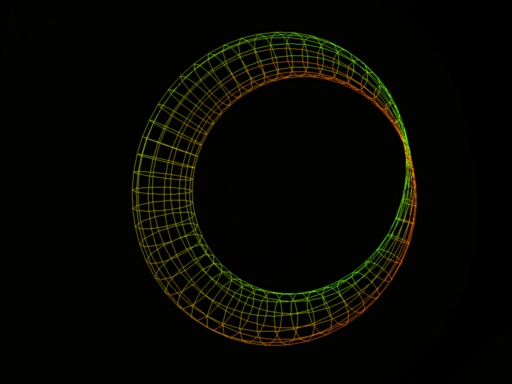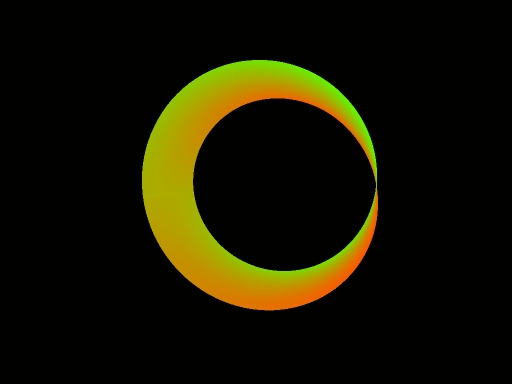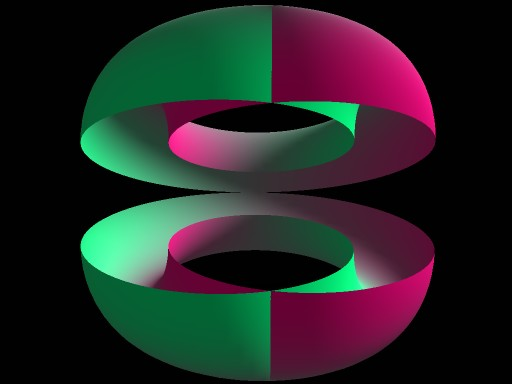Klein Bottle
I were thinking about various geometric surfaces with Klein bottle topology, such as the most famous one(that looks like bottle), figure 8, and others. (there you can see good collection)
and have discovered (or likely rediscovered) this elegant 4D surface with Klein bottle topology:

eq 1;
x=sin(α)*(1+a*sin(β)*cos(0.5*α))
y=cos(α)*(1+a*sin(β)*cos(0.5*α))
z=a*cos(β)
w=sin(0.5*α)*a*sin(β)

(a=0.5 works fine. It defines thickness of tube)
Its a pinched torus Klein bottle 5, naturally extended to four dimensions.

There is some screenshots from OpenGL visualization, using xyz as coordinate of the point of surface and using w as color (from green to red)Doesn't look much like bottle eh?
In 4 dimensions, surface does not intersect itself - the surfaces on "intersection" have different colors and thus are far apart in the 4th dimension. The "intersection" corresponds to 4-dimensional rotation, where thickness of the torus turns into w coordinate and then turns back into thickness that becomes negative (i.e. mirrored).
Flatland analogy is flipping flatland object "upside down". To flatlanders that will look like mirroring.
You can see it better on this image where surface has been cut in half to show interior:Each half is just twisted Moebius strip in 4D that in this projection appears to intersect itself. If we swap Z and W, i.e. look at essentially same thing with different 4D-->3D projection, two Moebius strips that corresponds to halves of the bottle will be more obvious:(cut into halves same way, other projection)
By color you can see that one strip "bends" into +w direction (i.e. central part of strip has more intense color) and other is bended into -w, so when combined them form full "Klein bottle".
Eq 1 is closely related to the "traditional" Moebius strip(1) surface:

eq 2;
x=sin(α)*(R+s*cos(0.5*α))
y=cos(α)*(R+s*cos(0.5*α))
z=sin(0.5*α)*s
0<=α<2*π
-a<=s<a

, if you substitute s=a*sin(&beta) and let R=1, you get 3 coordinates (x,y,w) of eq 1.

My little interactive viewer(improved since taking the screenshots above):
klein3.exe
sdl.dll
controls:
a,s: accelerate, decelerate
spacebar: stop
mouse: rotate camera
d,f: tilt
1,2,3,4: different rendering modes (1 and 2 with backface culling, though because this surface has no inside and no outside, backface culling looks visually confusing)
r: reset 4D rotation of surface.
v,b,n,m: 4D rotation of surface. You need to press two keys simultaneously to specify what axises to rotate, and pressing order (i.e. which key is pushed first) defines the direction of rotation. Example, pressing "v" then "b" will rotate x and y in one direction(in 3D you would name that "rotate around z axis", but 3D is special case, more below). b and then v will rotate in opposite direction. v and m will rotate x and w , and the surface will appear to morph and change colors. (actually it does not morph; that's how rotation into w looks)
By pressing n and m you rotate z and w, this way you can get sorta Moebius strip.

Generally speaking, rotations is defined by pair of axises. In 2D space there is one pair, x and y, thus rotation is specified by single angle. In 3D there is (3*2)/2= 3 pairs, same as number of dimensions so you can talk about rotation "around axis". But it is not so in 4D where you have (4*3)/2=6 pairs, i.e. 6 degrees of rotational freedom (total 10 DOF). The "press two keys out of N" scheme is most general way, also it is definately better than 12 keys (6 for clockwise and 6 for counterclockwise)

Screensaver-ish version of same program: klein2.exe
(4D rotation happens without user input.)

REFERENCES:
1:
Eric W. Weisstein. "Moebius Strip." From MathWorld--A Wolfram Web Resource.
http://mathworld.wolfram.com/MoebiusStrip.html
2:
Eric W. Weisstein. "Klein Bottle." From MathWorld--A Wolfram Web Resource.
http://mathworld.wolfram.com/KleinBottle.html
3:
Geometry Center / Thomas Banchoff. "The Klein Bottle in Four-Space."
http://www.geom.uiuc.edu/~banchoff/Klein4D/Klein4D.html
4:
Konrad Polthier. "Imaging maths - Inside the Klein bottle."
http://plus.maths.org/issue26/features/mathart/index-gifd.html

Strangely enough, I can not find anything like eq1 surface in the web - only bottle-like Klein bottles, figure8's and some more exotic stuff.
Update: One of readers has pointed me to Pinched Torus klein bottle, as seen e.g. on
5:
Berthard Steven, Dolle Ethan. "Surface Constructions"
http://math.arizona.edu/~ura/013/bethard.steven/construct.html